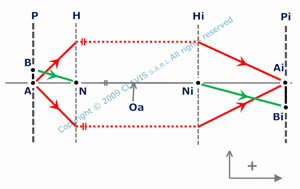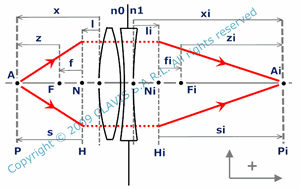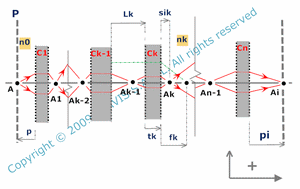and ortutorials

# paraxial conjugation

Optical system

In geometrical optics, optical systems are assemblies of optical components for redirecting the light in a controlled manner. Many of them are used to create the image of a real object in order to bring it closer, better discern its details, project it on a screen, etc. ... Each point of the object have a "conjugated" point in the image through the optical system. This means that the optical system transforms all light rays from an object point into light rays focused to its conjugated point in the image.

Gauss conditions

It is assumed that optical systems considered have a symmetry of revolution around an axis called the optical axis. Most optical systems are not rigorously stigmatic, which means that for incident light rays coming from an object point, the optical system does not produce emerging light rays converging rigorously to an image point. However, for object points close enough to the optical axis and light rays direction close enough to the optical axis direction, emerging light rays may converge approximately to an image point ( called the paraxial image ). It is said that the optical system gives an approached stigmatism and is working in Gauss conditions. This approached stigmatism is also called paraxial conjugation.

Transverse magnification

For optical systems working in Gauss conditions, the paraxial image Pi of a plane P perpendicular to the optical axis is also a plane perpendicular to the optical axis.Accordingly, for a segment made of two points A and B in the plane P, the conjugated segment AiBi is contained in Pi and is perpendicular to the optical axis. The transverse magnification m ( currently called "magnification" ) is the ratio AiBi/AB where AB and AiBi are the algebric lengths of the considered segments. Consequently, m is an algebric value.
m is constant whatever the points A and B in the plane P.
It is negative when AB and AiBi have opposite signs (which means that object and image are oriented in opposite directions).
It is positive when AB and AiBi have the same sign ( which means that object and image are oriented in the same direction ).

m depends on the paraxial parameters of the optical system ( focal lengths, principal planes location ) and P position.

Position of the conjugated point

The position of the paraxial image Ai of an object point A on the optical axis is often given by si, the algebric distance from the secondary nodal point Ni to Ai. si depends on s, f ( or fi ), n0 and n1.

• s is the algebric distance from the primary nodal point N to A
• f and fi are the effective focal lengths respectively in the object and in the image space
• n0 and n1 are the refraction indexes respectively in the object and in the image space
Ai position can also be calculated with xi, the algebric distance from the vertex of the last optical surface to Ai.
xi is a function of x, f ( or fi ), n0, n1, l and li.
• x is the algebric distance from the vertex of the first optical surface to A
• l is the algebric distance from the first optical surface vertex to the primary nodal point N
• li is the algebric distance from the last optical surface vertex to the secondary nodal point NiSometimes, it can be useful to calculate z and zi which are respectively the algebric distances FA and FiAi ( F and Fi being respectively the front and back focal points ).

In some cases, it is not convenient to determine paraxial parameters of the whole optical system for calculating Ai position and magnification m.
Indeed, for a simple optical component as a dioprum, xi can be directly calculated with x, surface radius R and media indexes n0 and n1. For a spherical mirror, xi is a simple function of x and mirror radius R.For a system made by a combination of n optical components C1, C2,, Cn ( like lenses, mirrors,  ), Ai is the conjugated of An-1 by Cn, An-1 is the conjugated of An-2 by Cn-1 , Ak is the conjugated of Ak-1 by Ck , , A1 being the conjugated of A by C1. Ai position may be determined by calculating the position of each intermediate points Ak.

• sik is the algebric distance from the secondary principal plane of Ck to Ak
• p is the algebric distance from the primary principal plane of C1 to A
• Lk is the distance from the secondary principal plane of Ck-1 to the primary principal plane of Ck
• tk is the distance from the primary principal plane to the secondary principal plane of Ck
• fik is the effective focal length in the image space of Ck
• nk is the refraction index of the medium following Ck
• n0 is the refraction index in the object space
pi giving the position of Ai regarding the secondary principal plane of Cn depends on p and n0, but also on sik, tk, fik, nk ( with k from 1 to n ) and Lk ( with k from 2 to n ).

For example , it may be practical to calculate Ai position and magnification m by calculating intermediate images for an assembly of two thin lenses, as well as for a catadioptric system made of a thin lens and spherical mirror.

Numerical apertureAll optical systems have a limited aperture size imposed by an optical surface or a diaphragm... It limits the number of light rays ( and consequently the light power ) entering in the optical system. Therefore, considering an object point A, the numerical aperture in the object space NA characterizes the proportion of light rays coming from A and potentially entering by the optical system. Likewise, the numerical aperture NAi in the image space characterizes the proportion of light rays focusing on Ai and potentially coming out of the optical system.

NA = n0.sin (alfa) and NAi = n1.sin (alfai).

n0 and n1 are the refraction indexes respectively in the object space and in the image space. alfa and alfai are the angles of the marginal light rays with the optical axis respectively in the object space and in the image space. In the case where the aperture has a diameter D and is located in the primary or secondary principal plane, NA depends on s, D and the refraction index ( n0 ) of the object space. NAi depends on si, D and the refraction index ( n1 ) of the image space.

Depth of field

In many cases, an optical system is dedicated to form the image of an object in a plane that can be called "observation plane". This plane can contain, for example, the active area of a detector. When the detector is supposed to detect a ponctual image, its active area has to be as small as possible. However, it can not be ponctual. It makes no difference when detecting a ponctual image or a spot image smaller than the active area. Consequently, in a certain limit, active area can be out of the image plane, giving therefore some tolerance on object or observation plane positions. This tolerance is commonly called depth of field or defocus depending if the object or observation plane positioning is considered. More generally, there is a maximum spot size acceptable in the observation plane which defines a certain depth of field and defocus.For an optical system working in Gauss conditions, the depth of field ( ds ) as well as the defocus ( dsi ) depend on :

• the effective focal length in the image space( fi ),
• the aperture diameter ( D ),
• the distance from the primary principal plane to the object ( s ),
• the maximum image spot diameter authorized ( Dr ).References

"Optique Fondements et applications" - 2004 - author : José-Philippe Perez.

"Optique géométrique Imagerie et instruments" - 2007 - author : Bernard Balland.

"Optique géométrique paraxiale" - Institut d'optique théorique et appliquée - 1985 - author : Michel Cagnet.

"Formation des images Aberrations" - Institut d'optique théorique et appliquée - 1985 - author : Michel Cagnet.